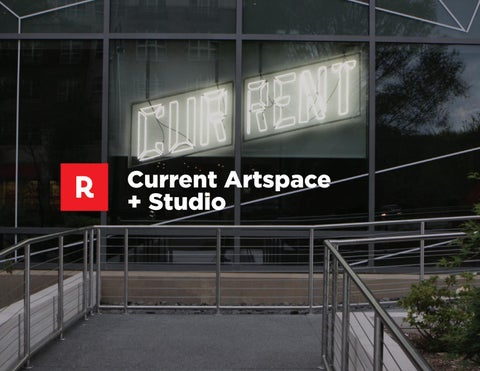Current Artspace + Studio

CU R R E NT A R T S PAC E + STU D I O P R E - L AU N C H

CU R R E NT A R T S PAC E + STU D I O P R E - L AU N C H

CU R R E NT A R T S PAC E + STU D I O P R E - L AU N C H

CU R R E NT A R T S PAC E + STU D I O L AU N C H

CU R R E NT A R T S PAC E + STU D I O B U S WR A P S

XXXX

10

STUDENT

TICKETS

currentunc.org

OPEN NOW

A AT C

NA ROLI

SQU

ARE

!

POWERED BY CAROLINA PERFORMING ARTS

Connect with us!

